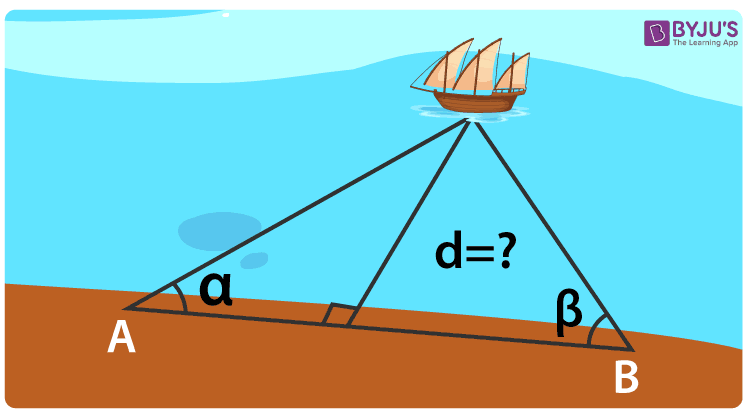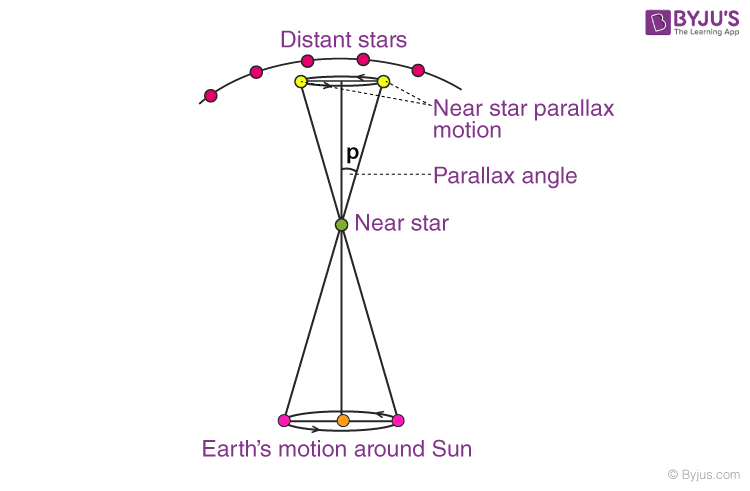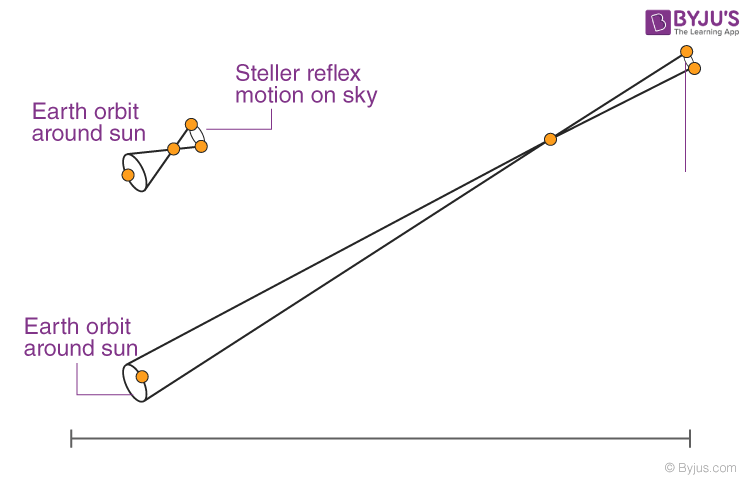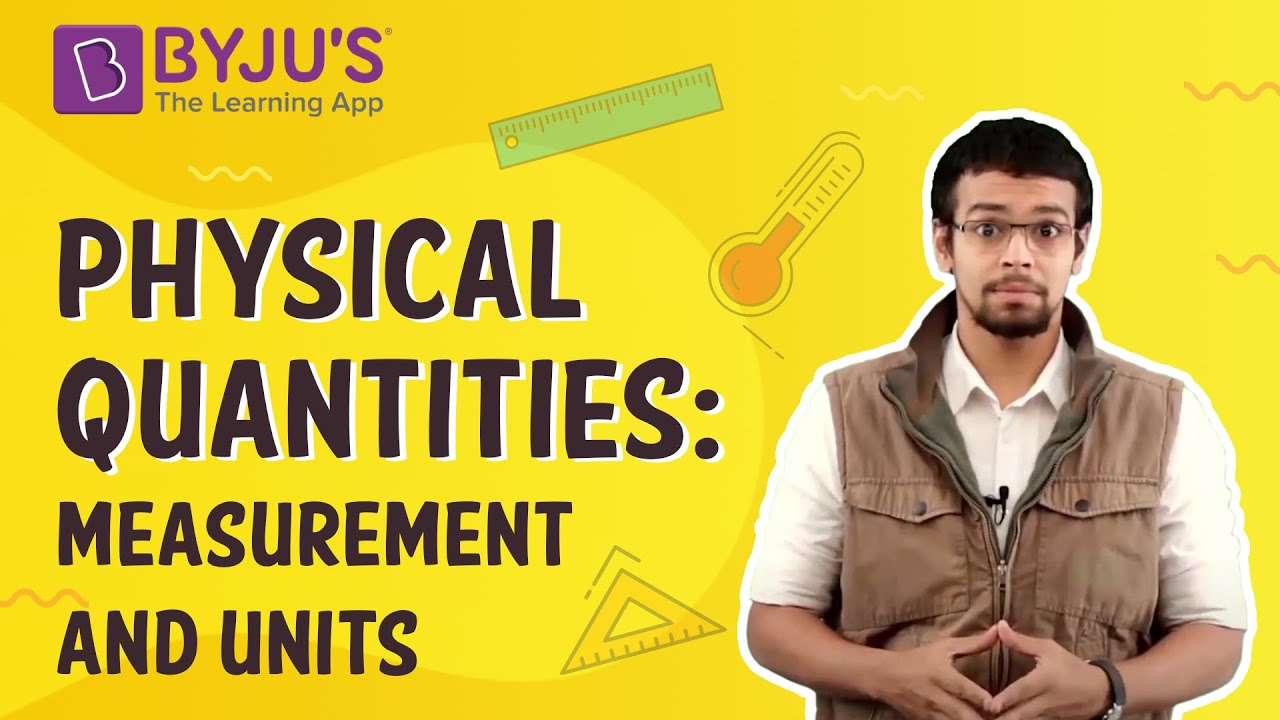# Measurement of Length : Triangulation and Parallax Method

In day-to-day life, measurement of length is unavoidable. Small distances can be measured using the scale and expressed using a meter or foot. Large distances like the distance between two cities or two countries are measured using maps and electronic devices and expressed using kilometres or miles. Now, going a step ahead for measuring the distance between two stars, one needs efficient mathematical skills and techniques like – Triangulation and Parallax Method. The obtained values are expressed in light-years or astronomical units.

## Measuring Distance by Triangulation

Let’s try to understand what we mean by the triangulation method? How can triangulation help us measure the distances of faraway stars? The parallax method uses the fact that a triangle can be described completely with only three elements. This method of finding the triangle’s values to yield the location of an object is termed Triangulation.

Surveyors and architects regularly use such methods. Triangulation is the process of determining the location of a point by measuring the angles to it from two known points rather than measuring distances directly.

### Triangulation Example

Let’s try to apply this in a real-life example. How might we measure the distance of a ship from the shore without actually measuring it? It can be measured using the method of triangulation.Triangulation method of distance measurement

• Let’s create a fixed baseline with two different points AB.
• The angle extended by point A to the ship can be denoted as α (BAC) and the angle extended by B to the ship is denoted by a β.
• Now the baseline AB is known and so are the angles and hence we can deduce the rest of the properties of a triangle such as the position of the third point which is the ship.

## Parallax Method

Parallax is the displacement or change in the object’s apparent position when viewed from two different points of view. The two points of view have their own line of sight, and parallax is measured as half of the angle between the two lines of sight.

When you are travelling in a vehicle and looking around as you are moving, you will see that objects in the distance appear to move more slowly than the objects closer to you. This is the effect of parallax. Nearby objects have a larger parallax than distant objects, so the parallax can be used to determine distances.

The phenomenon of parallax, when combined with triangulation, will yield the location of the object with considerable accuracy. Astronomers regularly use the parallax method to measure the distances of closer stars.

### Distance Measurement by Parallax

Distance measurement by parallax is a special application of the principle of triangulation. We knew that triangles could be described completely if two angles and sides were known from triangulation.In the image shown above, the distance of a distant star is calculated. The star is closer to earth than the faraway stars and exhibits a finite parallax value. This way, we can obtain the value of the parallax angle by viewing the star from two known points on Earth, forming the baseline of the triangle.

Let’s define the parallax half-angle from two distinct points on Earth as ‘p’. Here the maximum value of‘ ‘d’ is the radius of Earth, and the star’s distance can be assumed to be only slightly farther than the sun. Here the value of the distance from the sun is many magnitudes smaller than the radius of the earth, due to which the value of the parallax angle we get is minimal. Too small to be even detected.

Cassini’s theory was correct, but the least count of the equipment used then wasn’t small enough. This is why the great Astronomer Cassini and his colleagues were unsuccessful in finding the parallax for even a single star. His detractors considered this to be proof that heliocentricity was wrong.

## Application

Assuming the angle ‘p’ is small, the distance to the object measured in parsecs (in terms of speed of light) is equal to the reciprocal of the parallax angle measured in arcseconds.

$$\begin{array}{l}D (parsec)= \frac{1}{p}(arcsec)\end{array}$$

To overcome the problem of the small ratios, star parallax is most often measured using annual parallax, which is defined as the difference in the position of a star as seen from the earth and sun. Instead of taking the fixed baseline as the Earth’s radius, the fixed baseline is taken as the radius of Earth’s revolution around Earth which increases the size of the baseline, therefore, the top angle making it easier to measure.Measurement of stellar distances and the parallax angle in terms of distancesUnfortunately, the ground-based telescopes can only measure parallax reliably for stars within a few hundred light-years from us. Telescopes above the atmosphere, such as the Hubble Telescope, can measure smaller parallax shifts and thus larger distance, but even in that case, the most distant objects for which distance can be determined by parallax of a few thousand light-years away.

Physics Related Articles:

## Frequently Asked Questions – FAQs

### What is Parallax?

Parallax is the displacement or change in the object’s apparent position when viewed from two different points of view. The two points of view have their own line of sight, and parallax is measured as half of the angle between the two lines of sight.

### What is Triangulation?

Triangulation is the process of determining the location of a point by measuring the angles to it from two known points rather than measuring distances directly.

### The parallax method is generally used for measuring the speed of light. True or False?

This is a false statement. Astronomers use the parallax method for measuring distances to the closest stars.

### What is the approximation made in the Parallax method?

In the parallax method, an approximation is made that the distance between a point on the earth and the planet is very large as compared to the distance between two points on the earth’s surface.

### The parallax method is used for measuring the distances of nearby stars only. Give reason.

Parallax method cannot be used for measuring very distant stars as in that case, the parallactic angle becomes too small to be measured accurately.

## Watch the video to find out what are base measurements